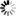Welcome to Central Library, SUST
Normal view

# Probability Models [electronic resource] / by John Haigh.

Material type:TextPublisher: London : Springer London : Imprint: Springer, 2013Edition: 2nd ed. 2013Description: XII, 287 p. 17 illus. online resourceContent type:
• text
Media type:
• computer
Carrier type:
• online resource
ISBN:
• 9781447153436
Additional physical formats: Printed edition:: No titleDDC classification:
• 519.2 23
LOC classification:
• QA273.A1-274.9
• QA274-274.9
Online resources:
Contents:
Probability Spaces -- Conditional Probability and Independence -- Common Probability Distributions -- Random Variables -- Sums of Random Variables -- Convergence and Limit Theorems -- Stochastic Processes in Discrete Time -- Stochastic Processes in Continuous Time -- Appendix: Common Distributions and Mathematical Facts.
Summary: The purpose of this book is to provide a sound introduction to the study of real-world phenomena that possess random variation. It describes how to set up and analyse models of real-life phenomena that involve elements of chance. Motivation comes from everyday experiences of probability, such as that of a dice or cards, the idea of fairness in games of chance, and the random ways in which, say, birthdays are shared or particular events arise. Applications include branching processes, random walks, Markov chains, queues, renewal theory, and Brownian motion. This popular second edition textbook contains many worked examples and several chapters have been updated and expanded. Some mathematical knowledge is assumed. The reader should have the ability to work with unions, intersections and complements of sets; a good facility with calculus, including integration, sequences and series; and appreciation of the logical development of an argument. Probability Models is designed to aid students studying probability as part of an undergraduate course on mathematics or mathematics and statistics.
Tags from this library: No tags from this library for this title.
Star ratingsAverage rating: 0.0 (0 votes)
No physical items for this record

Probability Spaces -- Conditional Probability and Independence -- Common Probability Distributions -- Random Variables -- Sums of Random Variables -- Convergence and Limit Theorems -- Stochastic Processes in Discrete Time -- Stochastic Processes in Continuous Time -- Appendix: Common Distributions and Mathematical Facts.

The purpose of this book is to provide a sound introduction to the study of real-world phenomena that possess random variation. It describes how to set up and analyse models of real-life phenomena that involve elements of chance. Motivation comes from everyday experiences of probability, such as that of a dice or cards, the idea of fairness in games of chance, and the random ways in which, say, birthdays are shared or particular events arise. Applications include branching processes, random walks, Markov chains, queues, renewal theory, and Brownian motion. This popular second edition textbook contains many worked examples and several chapters have been updated and expanded. Some mathematical knowledge is assumed. The reader should have the ability to work with unions, intersections and complements of sets; a good facility with calculus, including integration, sequences and series; and appreciation of the logical development of an argument. Probability Models is designed to aid students studying probability as part of an undergraduate course on mathematics or mathematics and statistics.

There are no comments on this title.